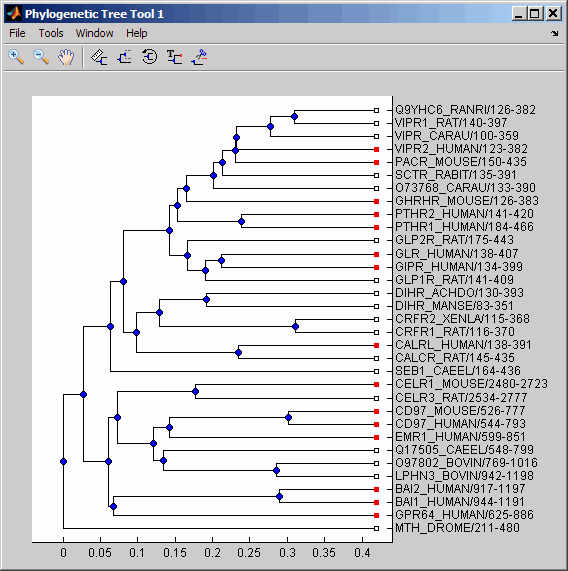# getbyname (phytree)

Branches and leaves from phytree object

## Syntax

```S = getbyname(Tree, Expression) S = getbyname(Tree, Key) S = getbyname(Tree, Key, 'Exact', ExactValue) ```

## Arguments

 `Tree` phytree object created by `phytree` function (object constructor) or `phytreeread` function. `Expression` Regular expression or cell array of regular expressions to search for in `Tree`. `Key` Character vector or cell array of character vectors to search for in `Tree`. `ExactValue` Controls whether the full exact node name must match the character vector(s), ignoring case. Choices are `true` or `false` (default). When `true`, `S` is a numeric column vector indicating which node names match a query exactly, in full.

## Description

`S = getbyname(Tree, Expression)` searches the nodes names in `Tree`, a phytree object, for the regular expression(s) specified by `Expression`. It returns `S`, a logical matrix of size `NumNodes`-by-`M`, where `M` is either `1` or the length of `Expression`. Each row in `S` corresponds to a node, and each column corresponds to a query in `Expression`. The logical matrix `S` indicates the node names that match `Expression`, ignoring case.

`S = getbyname(Tree, Key)` searches the nodes names in `Tree`, a phytree object, for the character vector(s) specified by `Key`. It returns `S`, a logical matrix of size `NumNodes`-by-`M`, where `M` is either `1` or the length of `Key`. Each row in `S` corresponds to a node, and each column corresponds to a query in `Key`. The logical matrix `S` indicates the node names that match `Key`, ignoring case.

```S = getbyname(Tree, Key, 'Exact', ExactValue)``` specifies whether the full exact node name must match the character vector(s), ignoring case. Choices are `true` or `false` (default). When `true`, `S` is a numeric column vector indicating which node names match a query exactly, in full.

## Examples

1. Read a phylogenetic tree file created from a protein family into a phytree object.

`tr = phytreeread('pf00002.tree');`
2. Determine all the mouse and human proteins by searching for nodes that include the character vectors `'mouse'` and `'human'` in their names.

```sel = getbyname(tr,{'mouse','human'}); view(tr,any(sel,2));```### Topics

Introduced before R2006a

## SupportGet trial now